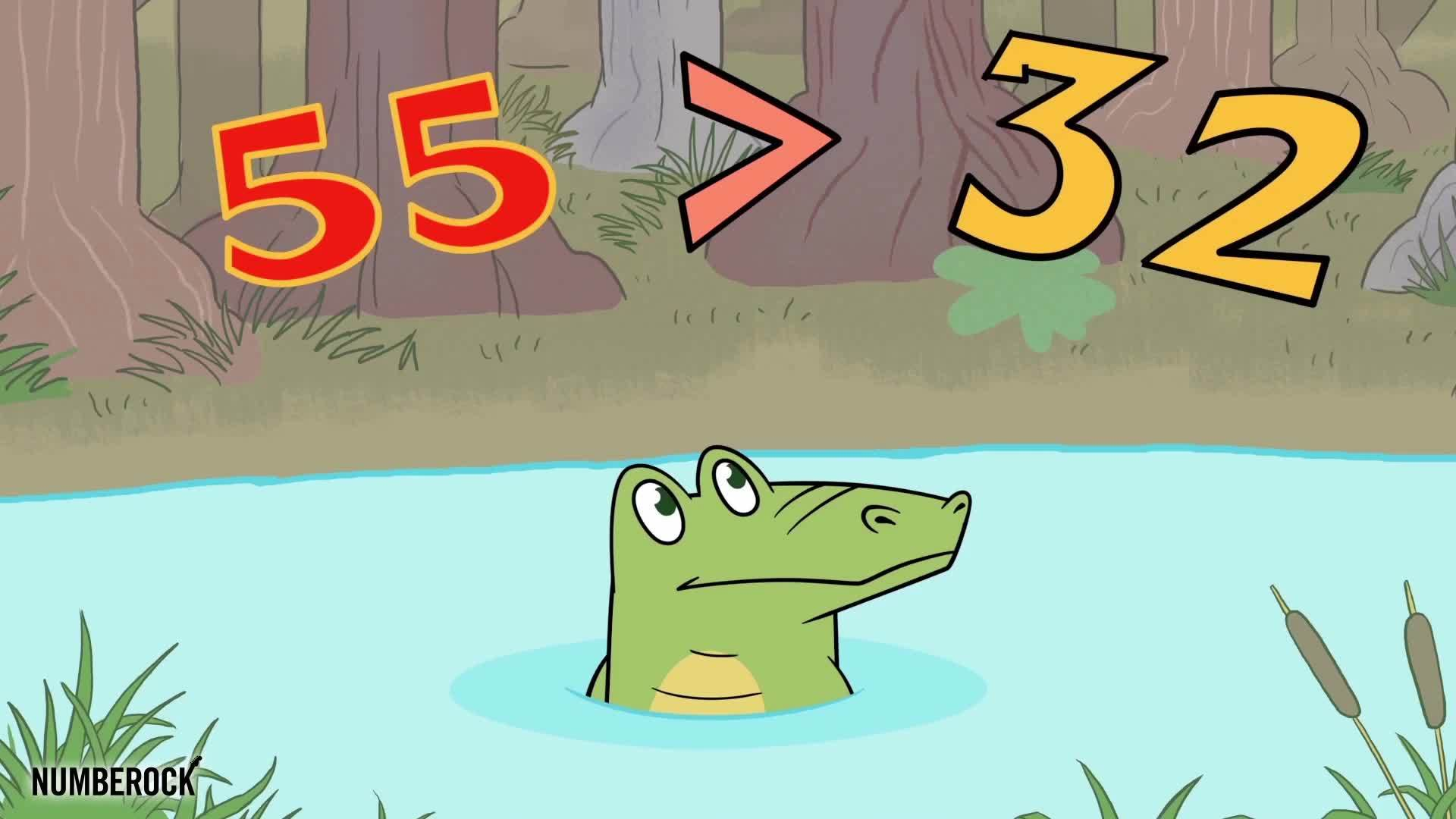# Greater Than Less Than Song for Kids | Comparing Numbers by Place Value

for ages
4
to
6
This video shows how to identify whether the number of objects in one group is greater than, less than, or equal to the number of objects in another group, e.g., by using matching and counting strategies. Understand that the two digits of a two-digit number represent amounts of tens and ones. Understand the following as special cases: 10 can be thought of as a bundle of ten ones — called a "ten." The numbers from 11 to 19 are composed of a ten and one, two, three, four, five, six, seven, eight, or nine ones.